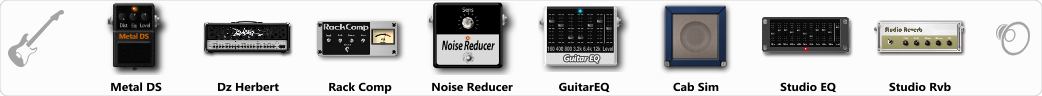# Swedish Death Metal 1

Discussion in 'ToneLib-GFX presets' started by Tetragramm1983, Jul 5, 2019.

1. ### Tetragramm1983Active Member

Swedish Death Metal 1

Preset name: Swedish Death Metal 1

Slighty wet on the verb. A good Tone

Effects chain:Effect: "Metal DS" (Overdrive / Distortion), active - "yes"
"Dist" = 45
"Bass" = 80
"Middle" = 65
"Treble" = 35
"Level" = 50

Effect: "Dz Herbert" (Amp simulators), active - "yes"
"Gain" = 45
"Bass" = 54
"Middle" = 35
"Treble" = 71
"Presence" = 85
"Master" = 85
"Output" = 50
"Level (dB)" = 4

Effect: "Rack Comp" (Dynamics / Filter), active - "yes"
"Threshold (dB)" = -24
"Ratio" = 4
"Attack" = Slow
"Release (ms)" = 300
"Knee" = 4
"Level (dB)" = 12

Effect: "Noise Reducer" (Dynamics / Filter), active - "yes"
"Sens" = 81
"Mode" = Hard

Effect: "GuitarEQ" (Dynamics / Filter), active - "yes"
"160 Hz" = -6
"400 Hz" = -10
"800 Hz" = -7
"1.6 kHz" = -2
"3.2 kHz" = -3
"6.4 kHz" = -3
"12 kHz" = -6
"Level (dB)" = 2

Effect: "Cab Sim" (Cabinets), active - "yes"
"Model" = 4x12" Cali v30
"Level (dB)" = 2

Effect: "Studio EQ" (Dynamics / Filter), active - "yes"
"31 Hz" = -15
"62 Hz" = -15
"125 Hz" = 3
"250 Hz" = -7
"500 Hz" = -8
"1 kHz" = -3
"2 kHz" = 0
"4 kHz" = 4
"8 kHz" = 4
"16 kHz" = -5
"above 16 kHz" = 0
"Level (dB)" = 1

Effect: "Studio Rvb" (Reverb), active - "yes"
"Time" = 1.9
"PreDelay" = 32
"LoDamp" = 0
"HiDamp" = 40
"Mix" = 35

Note: You will need to download and install the ToneLib-GFX software to use the preset.

#### Attached Files:

• ###### Swedish_Death_Metal_1.tlgfx
File size:
728 bytes
Views:
3,999
alienwaste666, MPM, imushliy and 7 others like this.
2. ### Tetragramm1983Active Member

Please vote and leave comments on all my tones... if possible... thank you!

3. ================================================== =============
ToneLib GFX (versión de firmware 3.0)
Celda preestablecida: 11A
Nombre predefinido: Death Metal sueco 1
Tempo de Bpm: sincronizado con el host

Efectos: {

Efecto: "Reductor de ruido" (Dinámica / Filtro), activo - "sí"
{
"Sens" = 53
"Modo" = Suave
}

Efecto: "Cent OD" (Overdrive / Distortion), activo - "yes"
{
"Drive" = 55
"Tono" = 54
"Nivel" = 38
}

{
"Ganancia" = 93
"Bajo" = 50
"Medio" = 50
"Agudos" = 50
"Presencia" = 50
"Maestro" = 50
"Nivel (dB)" = 3
}

Efecto: "Rack Comp" (Dinámica / Filtro), activo - "no"
{
"Umbral (dB)" = -24
"Relación" = 4
"Ataque" = Rápido
"Release (ms)" = 300
"Rodilla" = 4
"Nivel (dB)" = 0
}

Efecto: "GuitarEQ" (Dinámica / Filtro), activo - "sí"
{
"160 Hz" = 1
"400 Hz" = 0
"800 Hz" = -1
"1.6 kHz" = 2
"3.2 kHz" = 0
"6.4 kHz" = 5
"12 kHz" = 7
"Nivel (dB)" = 4
}

Efecto: "Cab Sim" (Gabinetes), activo - "sí"
{
"Modelo" = 1x12 "Blue Bell
"Nivel (dB)" = 2
}

Efecto: "Studio Rvb" (Reverb), activo - "yes"
{
"Tiempo" = 3.7
"PreDelay" = 32
"LoDamp" = 0
"HiDamp" = 40
"Mix" = 48
}

}; // Generado por ToneLib GFX ver. 3.9.8 (tonelib.net)
================================================== =============
una variación del preset